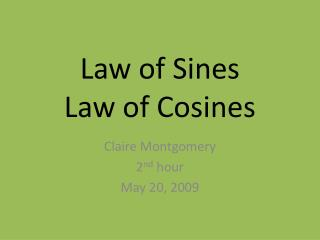DownloadDownload PresentationLaw of Sines Law of Cosines

# Law of Sines Law of Cosines

Download Presentation## Law of Sines Law of Cosines

- - - - - - - - - - - - - - - - - - - - - - - - - - - E N D - - - - - - - - - - - - - - - - - - - - - - - - - - -
##### Presentation Transcript

1. Law of SinesLaw of Cosines Claire Montgomery 2nd hour May 20, 2009

2. 6.1 Law of Sines • We use Law of Sines to solve oblique triangles. Oblique triangles are triangles with no right angle. • The angles of the triangle are always labeled A, B, and C. Their opposite side are labeled a, b, and c.

3. 6.1 Law of Sines • To solve an oblique triangle using Law of Sines you must know at least one side length and two other measurements. Either: • AAS or ASA – Two angles and a side • SSA – Two sides and an angle

4. Formulas • Law of Sine • Law of Sine reciprocal

5. Ambiguous Cases • If you are given two angles and one opposite side (SSA) three possible situations can occur • no triangle exists • one triangle exists • two distinct triangles exist

6. Ambiguous Case SSASample Problem • No triangle exists

7. Area of an Oblique Triangle • h = b sin A • Area = ½ (base)(height) = ½ (c)(b sin A) = ½ bc sin A • Area = ½ ab sin C = ½ ac sin B

8. Law of SineSample Problem • Solve the Triangle. Then find the area.

9. Law of SineStory Problem • A plane is flying in the air 160 ft from its starting point. It took off at an angle of 20 degrees. Its landing point is 300 ft from the starting point and it will land at an angle of 10 degrees. How many feet away is the plane currently from its landing point?

10. 6.2 Law of Cosines • To solve Oblique Triangles using Law of Cosines you again must know one side length and two other measurements. Either: • SSS – three side measures • SAS – two sides and their included angles

11. Formulas

12. Heron’s Area Formula • Use this formula to find the area of a triangle with Law of Cosines • Area =  s(s-a)(s-b)(s-c) • s = (a + b +c)/2

13. Law of CosineSample Problem • Solve the triangle. Then find the area using Heron’s formula 2 2 2 2

14. Law of CosineStory Problem • A bird is sitting on the top of the Leaning Tower of Pisa. It spots some food 20ft away from the base of the tower. How far will the bird have to fly if the Leaing tower of Pisa is 183.27 ft tall and leans at an angle of 4.00 degrees? c2 = a2 + b2 – 2(a)(b) cos C

15. Real World Application • People use Law of Sines and Law of Cosines everyday in construction, design, engineering, and architecture. • Mechanical engineers, architects, and math teachers use these laws all the time at their jobs. • An example of someone who can use these laws are firemen. If two stations get a call about a fire, they can use these laws to find out which station is closest to the fire. • If the two station know how far apart they are from each other and if they know how far they are both from the fire they could use Law of Cosine to calculate the angles at which they are from the fire and what angle the fire is from both of them. (SSS)

16. THE END